\$B:#>,\$N0lGU(B

\$BKhF|!":#>,\$N0lGU\$r>e\$2\$F\$\$\$^\$9!#(B
\$B:#>,0{\$`\$b\$N\$K:\$\$C\$?\$i!";29M\$K\$7\$F\$_\$F\$/\$@\$5\$\$!#(B

2021\$BG/(B3\$B7n(B

 1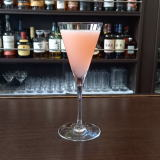(IJE16X(B 2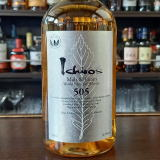(I2A[0=^(BM&G505 3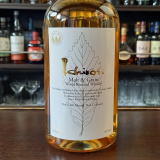(I2A[0=^(BM&G 4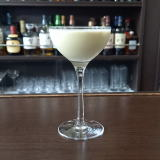(IL^Y%L[/8^(B 5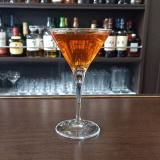(I?8WC=(B 6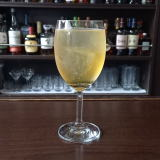(IK^1%=L_X/B'0(B 7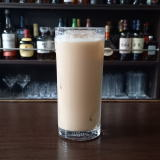(I=P=(B&(I3*/?](B 8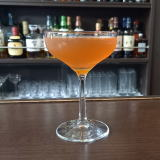(IL^W]C^0%<,]J_ZY(B 9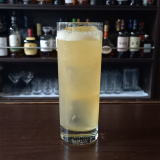(IO28%:X]=^(B 10 \$B5Y\$_(B 11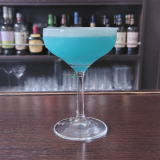(IL^Y0%ZC^((B 12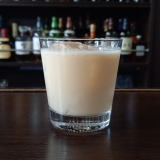(IP/D^E2D%63N^02(B 13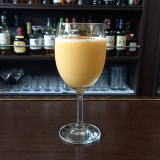(IN^=D]%LX/L_(B 14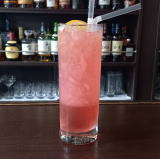(I[]8^%K^0A%C(0(B 15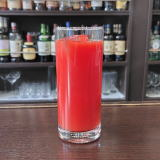(IL^W/C^(%;Q(B 16 \$B5Y\$_(B 17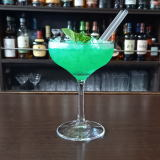(IP]D%LW/M_(B 18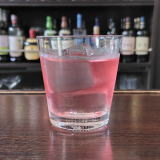(I=L_X]8^%J^0D^(B 19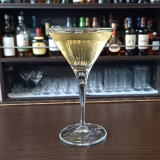(IF-0V060(B 20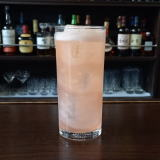(I;8W%L(=^(B 21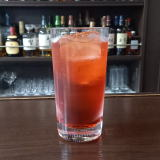(I<,0X0%C]L_Y(B 22(I<^.0<^1%P]D<^-Z/L_(B 23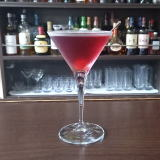(I1]E%6Z0FE(B 24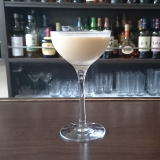(IJ^0J^X0%:0=D(B 25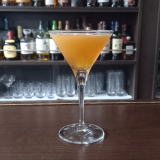(IF-0D]=^%=M_<,Y(B 26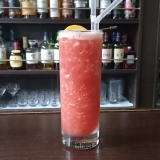(I<]6^N_0Y%=X]8^(B 27(IL^W]C^0%:L^W0(B 28 \$B5Y\$_(B 29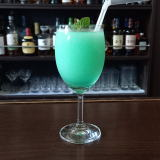(IL[0=^]%P]D%@^27X(B 30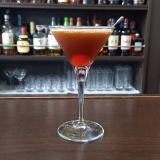(IF/8=%53](B 31(IN_0D\2]%;]6^X0(B